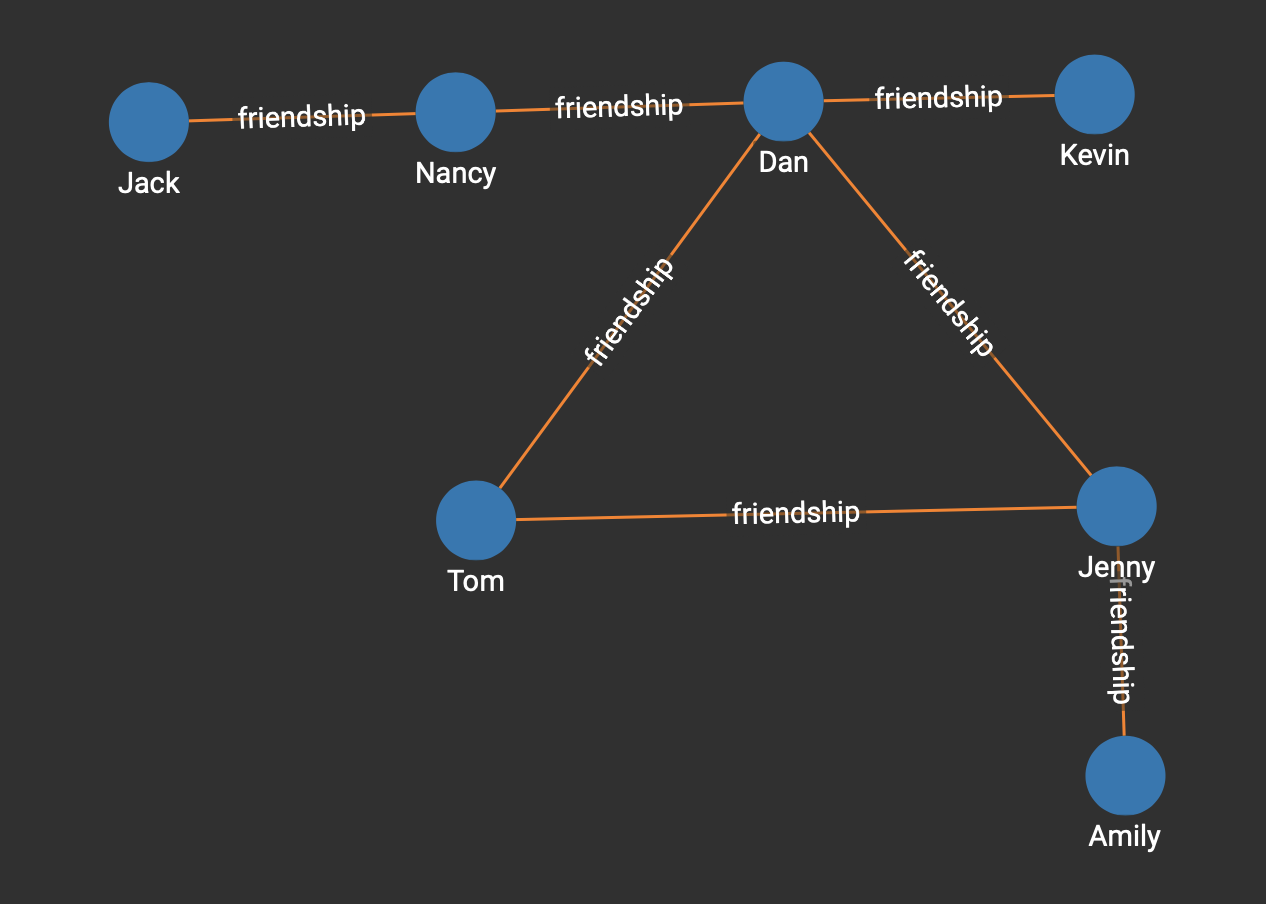# K-Core Decomposition

A k-core of a graph is a maximal connected subgraph in which every vertex is connected to at least k vertices in the subgraph. To obtain the k-core of a graph, the algorithm first deletes the vertices whose outdegree is less than k. It then updates the outdegree of the neighbors of the deleted vertices, and if that causes a vertex’s outdegree to fall below k, it will also delete that vertex. The algorithm repeats this operation until every vertex left in the subgraph has an outdegree of at least k.

Our algorithm takes a range of values for k and returns the set of the vertices that constitute the k-core with the highest possible value of k within the range. It is an implementation of Algorithm 2 in Scalable K-Core Decomposition for Static Graphs Using a Dynamic Graph Data Structure, Tripathy et al., IEEE Big Data 2018.

## Time complexity

O(E), where E is the number of edges in the graph.

## Specifications

``````tg_kcore(STRING v_type, STRING e_type, INT k_min = 0, INT k_max = -1,
BOOL print_accum = TRUE, STRING attr = "", STRING file_path = "",
BOOL show_membership = FALSE, BOOL show_shells=FALSE)``````

## Parameters

Parameter Description

`v_type`

Vertex type to include in the k-core

`e_type`

Edge type to count for k-core connections

`k_min`

Minimum value of k. If the actual maximum core is below `k_min`, the algorithm will return an empty set.

`k_max`

Maximum value of k. If `k_max` is smaller than `k_min`, the algorithm will ignore this parameter and keep looking for k-cores until it reaches a value of k where a k-core cannot be found.

`show_membership`

If `show_membership` is `true`, the algorithm will return the k-cores found for every value of k within the range provided. For each k-core, the results will include its member vertices.

`show_shells`

The *k**-shell* is the set of vertices that are part of the k-core but not part of the (k+1)-core. If `show_shells` is `true`, the algorithm will return the k-shells found for every value of k. within the range provided. For each k-shell, the results will include its member vertices.

`print_accum`

Boolean value that decides whether the algorithm will return output in JSON

`attr`

Optional. An attribute of the vertex to save the core level of the vertex to. If `attr` is provided, the core level of the vertex will be saved to this attribute of the vertex.

`file_path`

Optional. If `file_path` is provided, the algorithm will output results to a file specified by the file path in CSV format.

## Example

In the example below based on the `social` graph from GSQL 101, we can see that Dan, Tom, and Jenny make up a 2-core, which is the max-core of the graph:If we run the `kcore` algorithm on this small graph like so:

``RUN QUERY tg_kcore("person", "friendship", 0, -1, TRUE, "", "", FALSE, FALSE)``

Here is the returned JSON response, which includes a 2-core that is comprised of Dan, Jenny, and Tom:

``````[
{
"core_size": 3,
"k": 2,             // the k-core with the highest possible k is returned
"max_core": [
{
"attributes": {
"@core": 2,
"@deg": 0,
"age": 40,
"gender": "male",
"name": "Tom",
"state": "ca"
},
"v_id": "Tom",
"v_type": "person"
},
{
"attributes": {
"@core": 2,
"@deg": 0,
"age": 34,
"gender": "male",
"name": "Dan",
"state": "ny"
},
"v_id": "Dan",
"v_type": "person"
},
{
"attributes": {
"@core": 2,
"@deg": 0,
"age": 25,
"gender": "female",
"name": "Jenny",
"state": "tx"
},
"v_id": "Jenny",
"v_type": "person"
}
]
}
]``````